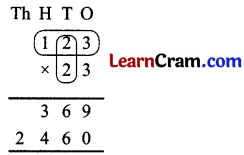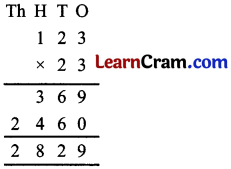# DAV Class 3 Maths Chapter 4 Worksheet 7 Solutions

The DAV Class 3 Maths Book Solutions and DAV Class 3 Maths Chapter 4 Worksheet 7 Solutions of Multiplication offer comprehensive answers to textbook questions.

## DAV Class 3 Maths Ch 4 WS 7 Solutions

Question 1.
234 × 28
Solution:
234 × 28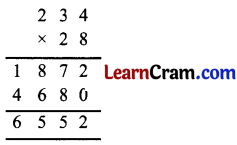Question 2.
453 × 16
Solution:
453 × 16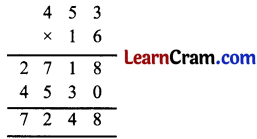Question 3.
223 × 38
Solution:
223 × 38Question 4.
272 × 25
Solution:
272 × 25Question 5.
153 × 36
Solution:
153 × 36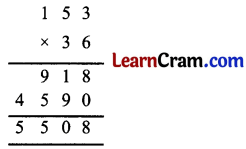Question 6.
205 × 23
Solution:
205 × 23Question 7.
162 × 42
Solution:
162 × 42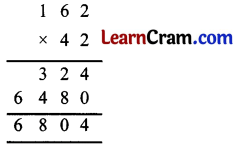Question 8.
356 × 48
Solution:
356 × 48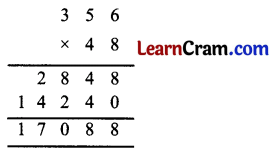Question 9.
275 × 29
Solution:
275 × 29Question 10.
312 × 68
Solution:
312 × 68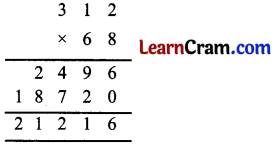Question 11.
112 × 63
Solution:
112 × 63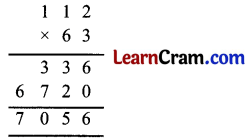Question 12.
350 × 47
Solution:
350 × 47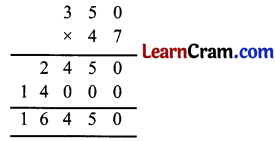### DAV Class 3 Maths Chapter 4 Worksheet 7 Notes

Multiplication (3 – digit numbers by 2 – digit numbers)

First multiply 123 by 3 ones

123 × 3 = 369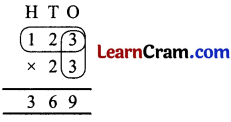Next, multiply 123 by 2 tens

123 × 2 = 246